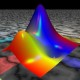Ask Your Question

# Discontinuous surface color by z-levelHi there, I want to plot a surface $z=f(x,y)$ together with the level curves (side by side). I know how to doi it when $f$ is continuous, or even bounded, but I run in trouble for uglier functions. Here are two examples (in CoCalC : a few things need to be adapted to use Jupyter) : the first works

var('x,y,s,t')
h(x,y)= x*y^2/(x^2+y^4)
cm = colormaps.Blues
def c(x,y):
return 0.6+x*y^2/(x^2+y^4+0.005)# Colorier ceci pose des problèmes, à cause de la singularité.
S=plot3d(h,(x,-1,1),(y,-1,1),color = (c,cm), opacity=1, mesh=1)
show(S)
C=contour_plot(h, (x,-1, 1), (y,-1, 1),cmap='Blues',linestyles='solid', colorbar=True)
show(C,figsize=4)


While the second, below, does not work as I would like to

h(x,y)= y/(x^2+y^2)
cm = colormaps.Spectral
def c(x,y):
return float(y/(x^2 + y^2+0.005))# Colorier ceci pose des problèmes, à cause de la singularité.
S=plot3d(h,(x,-1,1),(y,-1,1),color = (c,cm), opacity=1, mesh=1)
show(S, frame_aspect_ratio=[20,20,1])
C=contour_plot(h,(x,-1.5,1.5),(y,-1.5,1.5), cmap = "Spectral",
contours = [-2,-1, -0.5,-0.25,0,0.25,0.5,1,2], colorbar = True,
axes = True,
labels = True, label_colors='black',
label_inline=True, label_fontsize=8,
gridlines = True, axes_labels=['$x$','$y$'])
show(C,figsize=8)


I tried a few things, among other, what can be found https://ask.sagemath.org/question/758...here (old post), but the discontinuity seems to cause some problems.

Suggestions?

edit retag close merge delete

## Comments

Color function must take values between 0 and 1.

def c(x,y):
return float(y/(x^2 + y^2+0.005)) % 1


## 1 Answer

Sort by » oldest newest most votedInstead of plot3d, you could use implicit_plot3d. This allows a better control of the min and max values of the z-coordinate. Please, check the following code:

var("x,y,z")
h(x,y)= y/(x^2+y^2)

cm = colormaps.Spectral
zmin, zmax = -2, 2
def c(x,y,z):
return float((h(x,y)-zmin)/(zmax-zmin))

S = implicit_plot3d(z==h, (x,-1,1), (y,-1,1), (z,zmin,zmax),
color = (cm,c), region=lambda x,y,z: x^2+y^2+z^2>0.001)
show(S, aspect_ratio=[2,2,1], viewer="threejs")

C=contour_plot(h, (x,-1.5,1.5), (y,-1.5,1.5), cmap ="Spectral",
contours = [-2,-1,-0.5,-0.25,0,0.25,0.5,1,2],
colorbar = True, axes = True,
labels = True, label_colors="black",
label_inline=True, label_fontsize=8,
gridlines=True, axes_labels=["$x$","$y$"])
show(C, figsize=8)


You can see the result in this SageCell

Observe that now the color function needs three arguments. It always takes values between $0$ and $1$, since the $z$ range is constrained to the interval [zmin,zmax]. Likewise, since the contours also vary between zmin=-2 and zmax=2, colors in the surface match those in the contour plot. Points not satisfying the condition given by the region key are excluded from the plot, which, in this case, are points lying in a small ball centered at the origin. This allows to avoid the singularity of the function $h$. Hope that helps.

more

## Your Answer

Please start posting anonymously - your entry will be published after you log in or create a new account.

Add Answer

## Stats

Asked: 2019-05-16 03:12:51 +0200

Seen: 138 times

Last updated: May 18 '19